Question

# A. Calculate the after-tax cost of debt. b. Calculate the cost of preferred stock. c. Calculate t...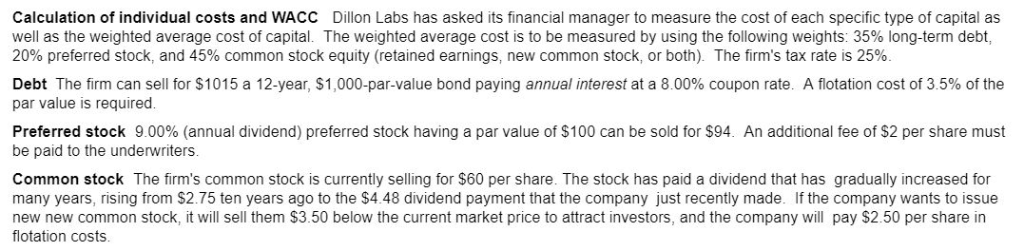a. Calculate the after-tax cost of debt.

b. Calculate the cost of preferred stock.

c. Calculate the cost of common stock (both retained earnings and new common stock).

d. Calculate the WACC for Dillon Labs.

Calculation of individual costs and WACC Dillon Labs has asked its financial manager to measure the cost of each specific type of capital as well as the weighted average cost of capital. The weighted average cost is to be measured by using the following weights: 35% long-term debt 20% preferred stock, and 45% common stock equity (retained earnings, new common stock, or both). The firm's tax rate is 25% Debt The firm can sell for \$1015 a 12-year, \$1,000-par-value bond paying annual interest at a 8.00% coupon rate. A flotation cost of 3.5% of the par value is required Preferred stock 9.00% (annual dividend) preferred stock having a par value of \$100 can be sold for \$94. An additional fee of \$2 per share must be paid to the underwriters Common stock The firm's common stock is currently selling for \$60 per share. The stock has paid a dividend that has gradually increased for many years, rising from \$2.75 ten years ago to the \$4.48 dividend payment that the company just recently made. If the company wants to issue new new common stock, it will sell them \$3.50 below the current market price to attract investors, and the company will pay \$2.50 per share in flotation costs

1.
=RATE(12,8%*1000,-1015*(1-3.5%),1000)*(1-25%)=6.207%

2.
=9%*100/(94-2)=9.783%

3.
Cost of common stock Using Retained earnings=4.48*(1+(4.48/2.75)^(1/10)-1)/60+(4.48/2.75)^(1/10)-1=12.841%

4.
Cost of common stock Using new common stock=4.48*(1+(4.48/2.75)^(1/10)-1)/(60-3.5-2.5)+(4.48/2.75)^(1/10)-1=13.712%

#### Earn Coins

Coins can be redeemed for fabulous gifts.

Similar Homework Help Questions
• ### a.  The​ after-tax cost of debt using the​ bond's yield to maturity​ (YTM) is The​ after-tax...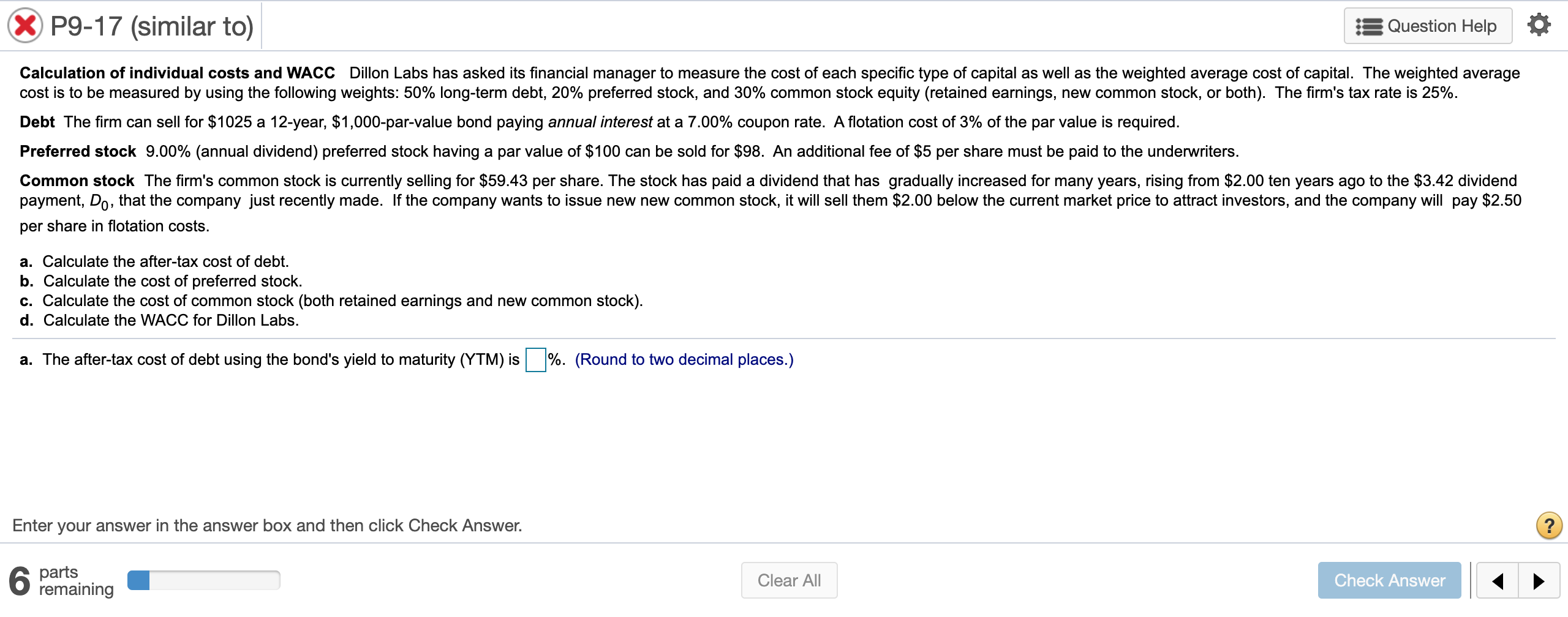a.  The​ after-tax cost of debt using the​ bond's yield to maturity​ (YTM) is The​ after-tax cost of debt using the approximation formula is b.  The cost of preferred stock is c.  The cost of retained earnings is The cost of new common stock is d.  Using the cost of retained​ earnings, the​ firm's WACC is Using the cost of new common​ stock, the​ firm's WACC is X P9-17 (similar to) Question Help Calculation of individual costs and WACC Dillon...

• ### Need assistance with A, B, C, D Calculation of individual costs and WACC Dillon Labs has...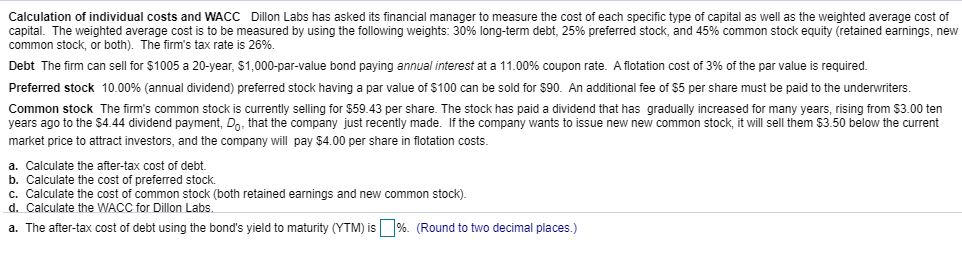Need assistance with A, B, C, D Calculation of individual costs and WACC Dillon Labs has asked its financial manager to measure the cost of each specific type of capital as well as the weighted average cost of capital. The weighted average cost is to be measured by using the following weights: 30% long-term debt, 25% preferred stock, and 45% common stock equity (retained earnings, new common stock, or both). The firm's tax rate is 26%. Debt The firm can...

• ### Dillon Labs has asked its financial manager to measure the cost of each specific type of...

Dillon Labs has asked its financial manager to measure the cost of each specific type of capital as well as the weighted average cost of capital. The weighted average cost is to be measured by using the following​ weights: 50 % ​long-term debt, 25 % preferred​ stock, and 25 % common stock equity​ (retained earnings, new common​ stock, or​ both). The​ firm's tax rate is 23​%. Debt: The firm can sell for ​\$1010 a 16 ​-year, ​\$1,000 ​-par-value bond paying...

• ### Dillon Labs has asked its financial manager to measure the cost of each specific type of...

Dillon Labs has asked its financial manager to measure the cost of each specific type of capital as well as the weighted average cost of capital. The weighted average cost is to be measured by using the following​ weights: 40​% ​long-term debt, 25​% preferred​ stock, and 35​% common stock equity​ (retained earnings, new common​ stock, or​ both). The​ firm's tax rate is 25​%. Debt The firm can sell for ​\$1010 a 19​-year, ​\$1 comma 000​-par-value bond paying annual interest at...

• ### Calculation of individual costs and WACC Dillon Labs has asked its financial manager to measure the...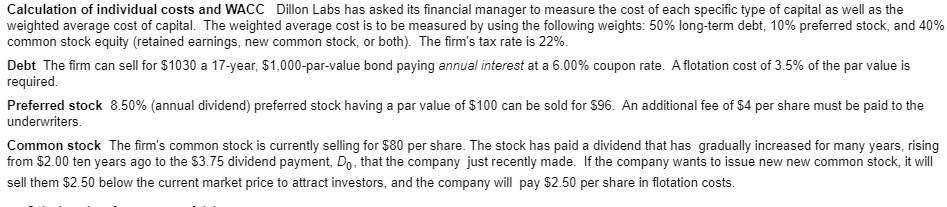Calculation of individual costs and WACC Dillon Labs has asked its financial manager to measure the cost of each specific type of capital as well as the weighted average cost of capital. The weighted average cost is to be measured by using the following weights: 50% long-term debt, 10% preferred stock, and 40% common stock equity (retained earnings, new common stock, or both). The firm's tax rate is 22%. Debt The firm can sell for \$1030 a 17-year, \$1,000-par-value bond...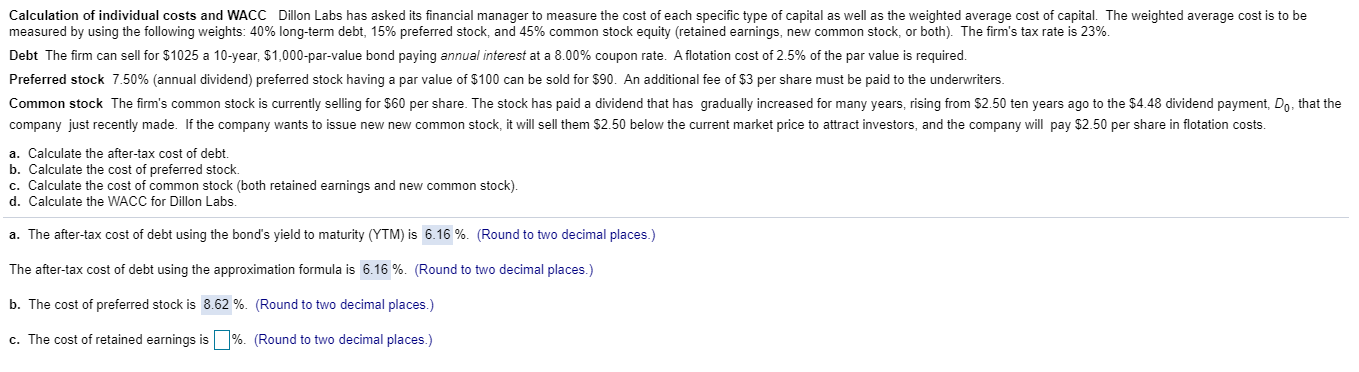Please help with part c & d! Show your work ! Thanks Calculation of individual costs and WACC Dillon Labs has asked its financial manager to measure the cost of each specific type of capital as well as the weighted average cost of capital. The weighted average cost is to be measured by using the following weights: 40% long-term debt, 15% preferred stock, and 45% common stock equity (retained earnings, new common stock, or both). The firm's tax rate is...

• ### Calculation of individual costs and WACC Dillon Labs has asked its financial manager to measure the...

Calculation of individual costs and WACC Dillon Labs has asked its financial manager to measure the cost of each specific type of capital as well as the weighted average cost of capital. The weighted average cost is to be measured by using the following weights: 40% long-term debt, 25% preferred stock, and 35% common stock equity (retained earnings, new common stock, or both). The firm's tax rate is 26%.Debt The firm can sell for \$1030 a 14-year, \$1,000-par-value bond paying...

• ### Dillon Labs has asked its financial manager to measure the cost of each specific type of capital as well as the weighted...

Dillon Labs has asked its financial manager to measure the cost of each specific type of capital as well as the weighted average cost of capital. The weighted average cost is to be measured by using the following​ weights: 40​% ​long-term debt, 25​% preferred​ stock, and 35​% common stock equity​ (retained earnings, new common​ stock, or​ both). The​ firm's tax rate is 22​%. Debt The firm can sell for ​\$1020 a 20​-year, ​\$1,000​-par-value bond paying annual interest at a 8.00​%...

• ### Please answer all parts of the question, thank you to anyone who helps me out! P9-17...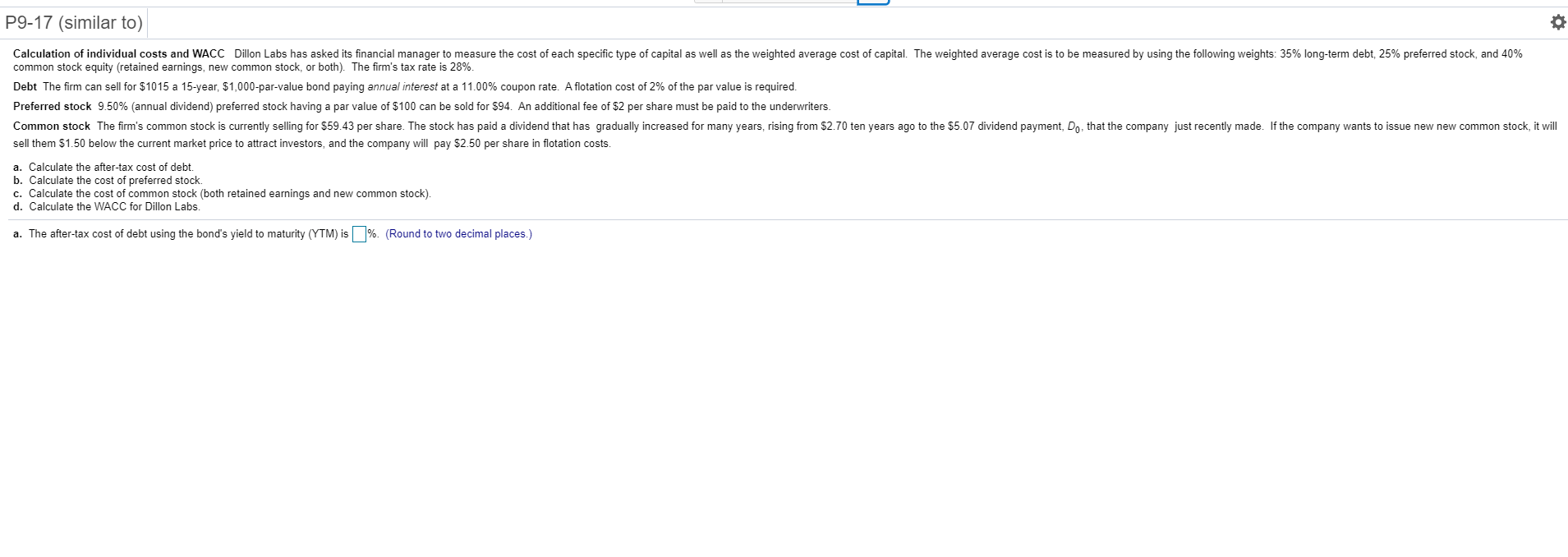Please answer all parts of the question, thank you to anyone who helps me out! P9-17 (similar to) 0 Calculation of individual costs and WACC Dillon Labs has asked its financial manager to measure the cost of each specific type of capital as well as the weighted average cost of capital. The weighted average cost is to be measured by using the following weights: 35% long-term debt, 25% preferred stock, and 40% common stock equity (retained earnings, new common stock,...

• ### Calculation of individual costs and WACC Dillon Labs has asked its financial manager to measure the...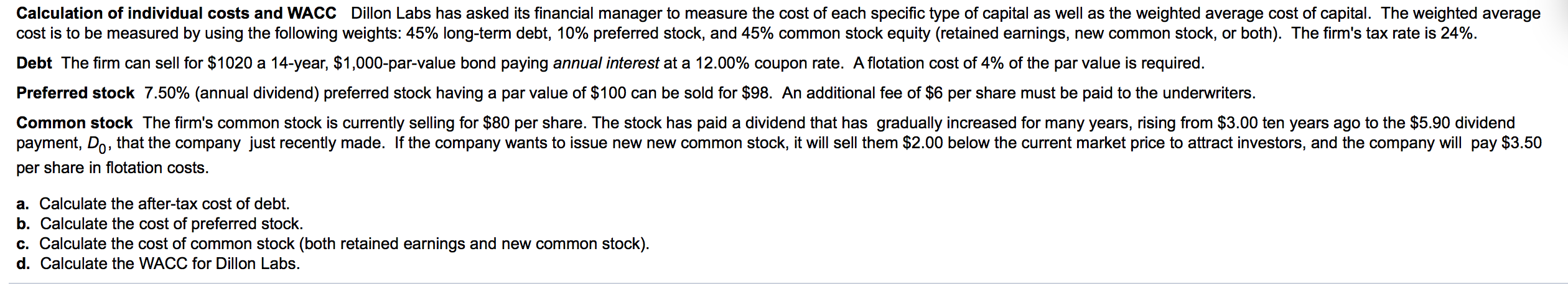Calculation of individual costs and WACC Dillon Labs has asked its financial manager to measure the cost of each specific type of capital as well as the weighted average cost of capital. The weighted average cost is to be measured by using the following weights: 45% long-term debt, 10% preferred stock, and 45% common stock equity (retained earnings, new common stock, or both). The firm's tax rate is 24% Debt The firm can sell for \$1020 a 14-year, \$1,000-par-value bond...